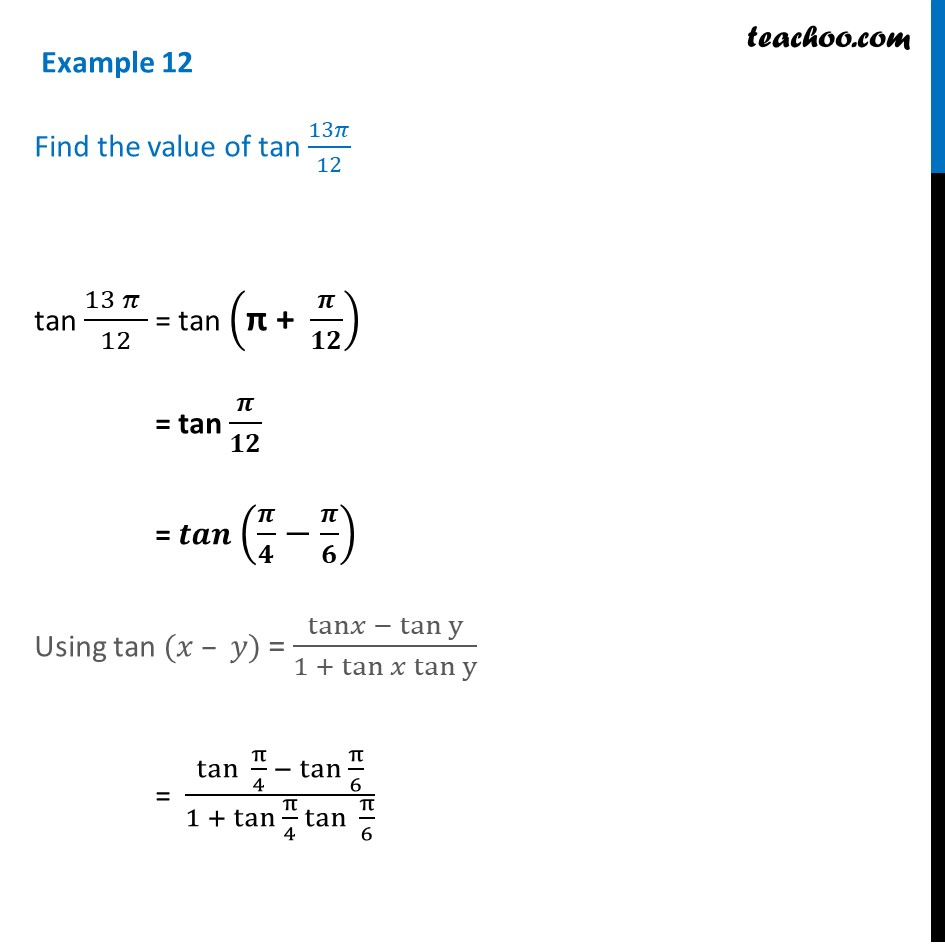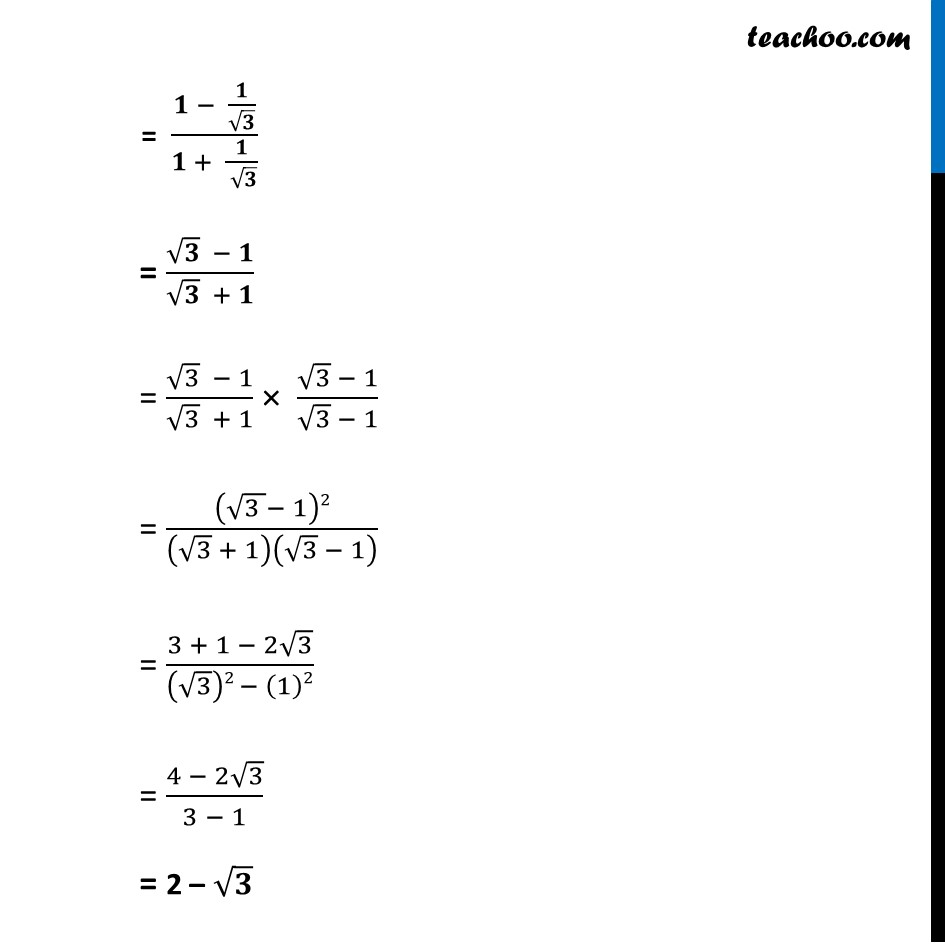Examples

Chapter 3 Class 11 Trigonometric Functions
Serial order wiseLearn in your speed, with individual attention - Teachoo Maths 1-on-1 Class

### Transcript

Example 12 Find the value of tan 13𝜋/12 tan (13 𝜋" " )/12 = tan ("π + " 𝝅/𝟏𝟐) = tan 𝝅/𝟏𝟐 = 𝒕𝒂𝒏 (𝝅/𝟒−𝝅/𝟔) Using tan (𝑥 – 𝑦) = (tan𝑥 − tan⁡y)/(1 + tan 𝑥 tan⁡y ) = (tan⁡〖 π/4〗 − tan π/6)/(1 + tan π/4 tan⁡〖 π/6〗 ) = (𝟏 − 𝟏/√𝟑)/(𝟏 + 𝟏/( √𝟑)) = (√𝟑 − 𝟏)/(√𝟑 + 𝟏) = (√3 − 1)/(√3 + 1) × (√3 − 1)/(√3 − 1) = (√(3 )− 1)2/(√3 + 1)(√3 − 1) = (3 + 1 − 2√3)/((√3)2 − (1)2) = (4 − 2√3)/(3 − 1) = 2 – √𝟑